# The Newsvendor Problem The most important questions of

• Slides: 15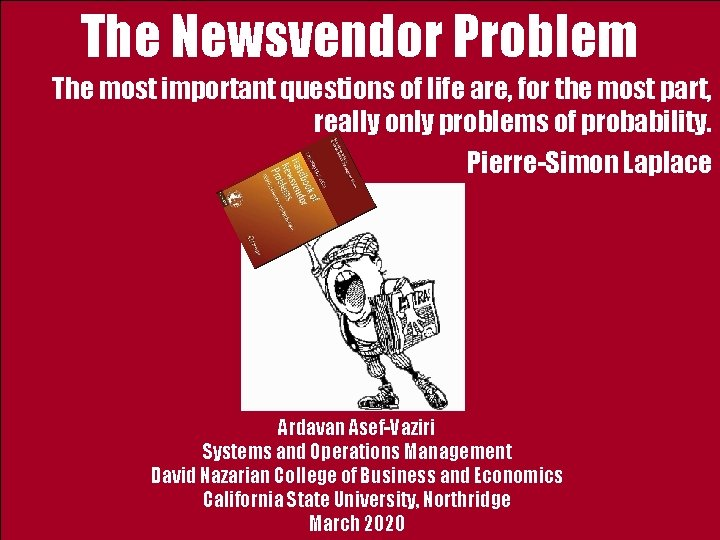The Newsvendor Problem The most important questions of life are, for the most part, really only problems of probability. Pierre-Simon Laplace Ardavan Asef-Vaziri Systems and Operations Management David Nazarian College of Business and Economics California State University, Northridge March 2020Teams and Clusters- In this Course and In Future Next Semester This Semester https: //youtu. be/_4 WLZ 8 j. Ux. Ic Basic Inventory Problems. A. Asef-Vaziri, March 2020. 2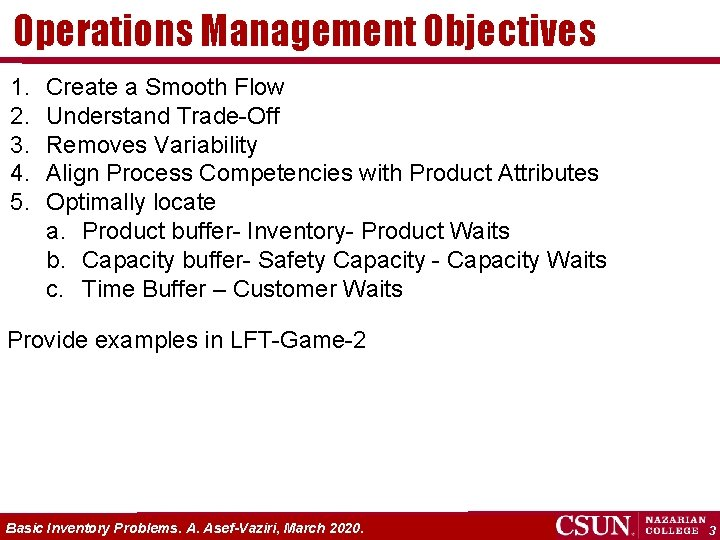Operations Management Objectives 1. 2. 3. 4. 5. Create a Smooth Flow Understand Trade-Off Removes Variability Align Process Competencies with Product Attributes Optimally locate a. Product buffer- Inventory- Product Waits b. Capacity buffer- Safety Capacity - Capacity Waits c. Time Buffer – Customer Waits Provide examples in LFT-Game-2 Basic Inventory Problems. A. Asef-Vaziri, March 2020. 3ROP-Multiple Choice-P 1 -P 2 1. Most inventory models attempt to minimize A) the number of items ordered and the safety stock B) total inventory costs and likelihood of a stockout C) the number of orders placed and the average inventory D) All of the above E) None of the above 2. Usage of the material is normally distributed with a mean of 20 pounds per day and a standard deviation of 4 pounds per day. Lead time is 4 days. What is the reorder point if the acceptable risk of a stockout is 1%? A) B) C) D) E) 90 86 20 99 102 Basic Inventory Problems. A. Asef-Vaziri, March 2020. 4Overage Cost vs. Underage Cost Swell Productions is sponsoring an outdoor conclave for owners of collectible and classic Fords. The concession stand in the T-Bird area will sell clothing such as official Thunderbird racing jerseys. Suppose the probability of jerseys sales quantities is Normally distributed with mean of 100 and standard deviation of 25. Suppose sales price is \$80 per jersey, purchase cost is \$40, and unsold jerseys are returned to the manufacturer for \$20 per unit. How many Jerseys Swell Production orders? Cu: Underage Cost Cu = 80 -40 = 40 Co: Overage Cost If Co was also 40 Blue Arrow (Blue Arrow + Red Arrow) = Cu/(Cu+Co) SL*= 40/(40+40) SL*= 0. 5 Basic Inventory Problems. A. Asef-Vaziri, March 2020. 40 40 5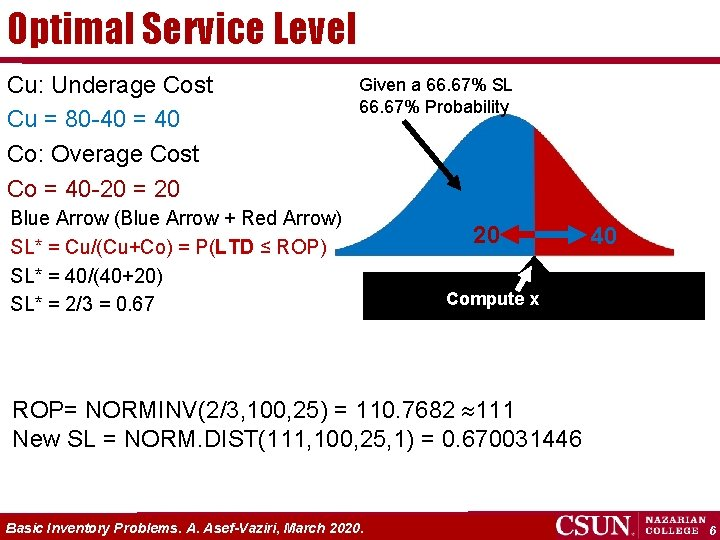Optimal Service Level Cu: Underage Cost Cu = 80 -40 = 40 Co: Overage Cost Co = 40 -20 = 20 Given a 66. 67% SL 66. 67% Probability Blue Arrow (Blue Arrow + Red Arrow) SL* = Cu/(Cu+Co) = P(LTD ≤ ROP) SL* = 40/(40+20) SL* = 2/3 = 0. 67 20 40 Compute x ROP= NORMINV(2/3, 100, 25) = 110. 7682 111 New SL = NORM. DIST(111, 100, 25, 1) = 0. 670031446 Basic Inventory Problems. A. Asef-Vaziri, March 2020. 6Problem 2. The News Vendor Problem An electronics superstore is carrying a 60” LEDTV for the upcoming Christmas holiday sales. Each TV can be sold at \$2, 500. The store can purchase each unit for \$1, 800. Any unsold TVs can be salvaged, through end of year sales, for \$1, 700. The retailer estimates that the demand for this TV will be Normally distributed with mean of 150 and standard deviation of 15. How many units should they order? Note: If they order 150, they will be out of stock 50% of the time. Which service level is optimal? 80%, 95%, 99%? ? Cost =1800, Sales Price = 2500, Salvage Value = 1700 Underage Cost = Marginal Benefit = p-c = 2500 -1800 = 700 Overage Cost = Marginal Cost = c-v = 1800 -1700 = 100 Optimal Service Level = SL* = P(LTD ≤ ROP) = Cu/(Cu+Co) Or in NVP Terminology SL* = P(R ≤ Q*) = Cu/(Cu+Co) Basic Inventory Problems. A. Asef-Vaziri, March 2020. 7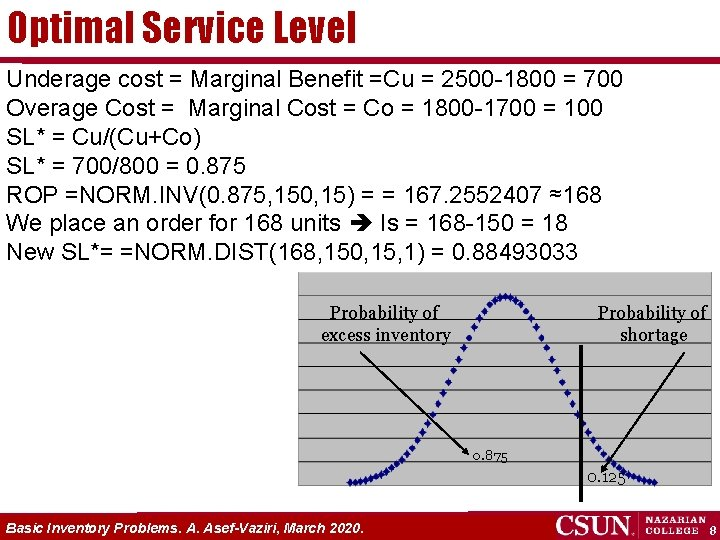Optimal Service Level Underage cost = Marginal Benefit =Cu = 2500 -1800 = 700 Overage Cost = Marginal Cost = Co = 1800 -1700 = 100 SL* = Cu/(Cu+Co) SL* = 700/800 = 0. 875 ROP =NORM. INV(0. 875, 150, 15) = = 167. 2552407 ≈168 We place an order for 168 units Is = 168 -150 = 18 New SL*= =NORM. DIST(168, 150, 15, 1) = 0. 88493033 Probability of excess inventory Probability of shortage 0. 875 0. 125 Basic Inventory Problems. A. Asef-Vaziri, March 2020. 8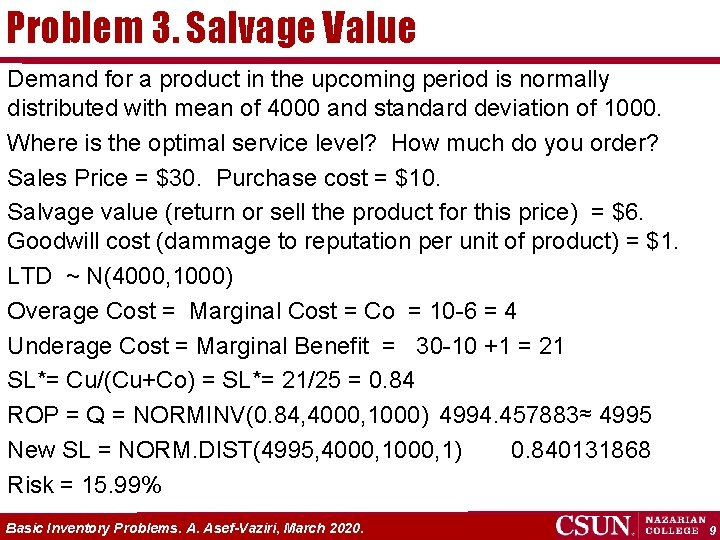Problem 3. Salvage Value Demand for a product in the upcoming period is normally distributed with mean of 4000 and standard deviation of 1000. Where is the optimal service level? How much do you order? Sales Price = \$30. Purchase cost = \$10. Salvage value (return or sell the product for this price) = \$6. Goodwill cost (dammage to reputation per unit of product) = \$1. LTD ~ N(4000, 1000) Overage Cost = Marginal Cost = Co = 10 -6 = 4 Underage Cost = Marginal Benefit = 30 -10 +1 = 21 SL*= Cu/(Cu+Co) = SL*= 21/25 = 0. 84 ROP = Q = NORMINV(0. 84, 4000, 1000) 4994. 457883≈ 4995 New SL = NORM. DIST(4995, 4000, 1) 0. 840131868 Risk = 15. 99% Basic Inventory Problems. A. Asef-Vaziri, March 2020. 9Problem 4. The Game Daily demand for your product R~N(R, R ) = N(20, 5). Sales price is \$100 per unit of product. You have decided to close this business line in 60 days. Your supplier has also decided to close hos line immediately, but has agreed to provide your last order at a cost of \$60 per unit. Any unsold product will be disposed at cost of \$10 per unit due to EPA regulations. How many units do you order LTD = R ×L =20 × 60 = 1200. Should we order 1200 units or more or less? If we order 1200, the chance of shortage/overstock is 50/50. Cu = 100 – 60 = 40 Co = 60 -0+10 =70 SL = Cu/(Cu+Co) = 40/(40+70) = 0. 3636. Basic Inventory Problems. A. Asef-Vaziri, March 2020. 10Less Than 50% Service Level Basic Inventory Problems. A. Asef-Vaziri, March 2020. 11The Newsvendor Problem Terminology In the NVP terminology, the following notations is used R: demand during the single period of study R =N(R, σR) Q* : What we order (only once) P(R ≤ Q*) = Cu/(Cu+Co) Basic Inventory Problems. A. Asef-Vaziri, March 2020. 12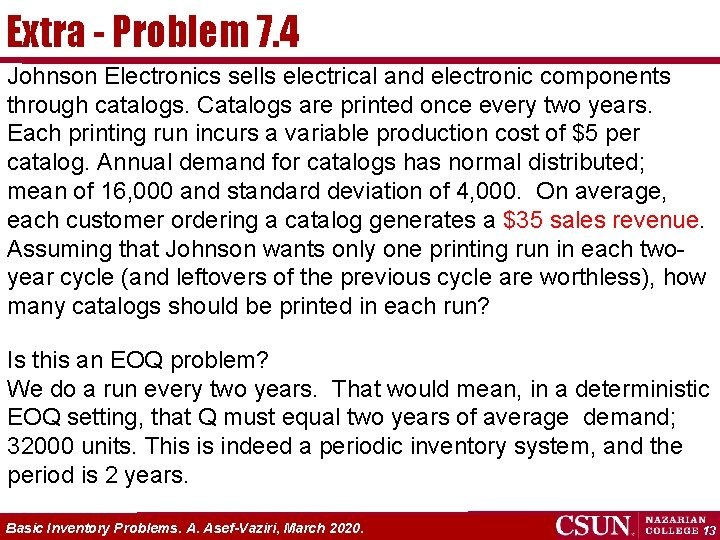Extra - Problem 7. 4 Johnson Electronics sells electrical and electronic components through catalogs. Catalogs are printed once every two years. Each printing run incurs a variable production cost of \$5 per catalog. Annual demand for catalogs has normal distributed; mean of 16, 000 and standard deviation of 4, 000. On average, each customer ordering a catalog generates a \$35 sales revenue. Assuming that Johnson wants only one printing run in each twoyear cycle (and leftovers of the previous cycle are worthless), how many catalogs should be printed in each run? Is this an EOQ problem? We do a run every two years. That would mean, in a deterministic EOQ setting, that Q must equal two years of average demand; 32000 units. This is indeed a periodic inventory system, and the period is 2 years. Basic Inventory Problems. A. Asef-Vaziri, March 2020. 13Extra - Problem 7. 4 The question is whether 32000 is the best quantity we can print every two years? Or we should print more. If we produce 32, 000 the probability of stockout is 50%. So this is an ROP problem when we want to have Isafety for 2 years. What the appropriate safety stock (or service level) should be. Every catalog fetches sales of 35 and costs 5 to produce. Cu = 35 - 5= 30. What is my overage cost? Each catalog costs \$5, if not used, salvages at the end of two year period. Co = 5 -0 =5 Basic Inventory Problems. A. Asef-Vaziri, March 2020. 14Extra - Problem 7. 4 Basic Inventory Problems. A. Asef-Vaziri, March 2020. 15